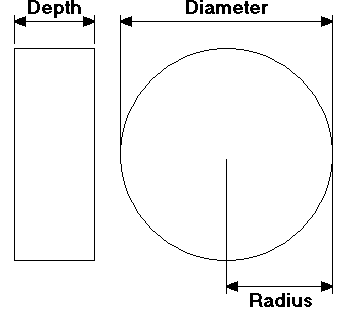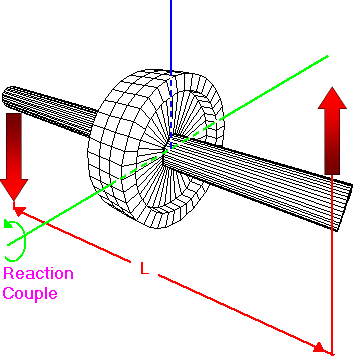MathsMore Maths# Gyroscope Math Page

(feedback welcome)
NOTE: You cannot edit the light coloured fields. This page requires Javascript.

### Notes and Reference Section

6.283185308 (approx) radians = 1 revolution
(2 * Pi radians = 1 revolution)

gyroscopic couple, C = I * W * Omega

Force on the bearings, F = (I * W * Omega) / L

 Basic Gyroscope MeasurementsDensity of material Radius (Meters) Diameter (Meters) (Radius * 2) Circumference (Meters) (2 * Radius * Pi) Depth (Meters) Volume (Meters Cubed) (Pi * (Radius ^ 2) * Depth) Mass (Kilograms) (Volume * Density) Pi Cut-Away Measurements Radius (Meters) Diameter (Meters) (Radius * 2) Circumference (Meters) (2 * Radius * Pi) Depth (Meters) Number of Cut-Aways Volume of Cut-Aways (Meters Cubed) (Pi * (Radius ^ 2) * Depth) Mass of Cut-Aways (Kilograms) (Volume * Density) Gyroscope Measurements(After Cut-Aways) Volume of Gyroscope (Meters Cubed) (Gyro volume - cutaways) Mass of Gyroscope (Kilograms) (Gyro mass - cutaways) Inertia Gyroscope Inertia (without cut-aways) (Mass * (radius ^ 2) / 2) Inertia Cut-Aways (Mass * (radius ^ 2) / 2) Omega (Gyroscope interia after cut-aways) (without cutaways - cutaways) Speed of Gyroscope W (radians per second) (rps * ((2 * pi)) Revolutions Per Minute (RPM) Kilometers Per Hour (KPH) (((rpm * circumference) / 1000) * 60) Degrees Per Second ((rpm * 360) / 60) Revolutions Per Second (rpm / 60) Speed of Main Structure Degrees Change Time in seconds Radians Change (degrees / (360 / (pi * 2)) Degrees Per Second (degrees / seconds) Revolutions Per Second (dps / 360) Revolutions Per Minute (RPM) (rps * 60) Omega (Radians per second) (rps * ((2 * pi)) Details of PrecessionC (Gyroscopic couple force) (Inertia * W * Omega) ForceDistance between bearings (Meters) Force on bearings (Newton Meters) (C / L) Force on each bearing (Newton Meters) ((C / L) / 2) Force on bearings (Kilograms) ((C / L) * 0.1) Force on each bearing (Kilograms) (((C / L) * 0.1 aprox) / 2)